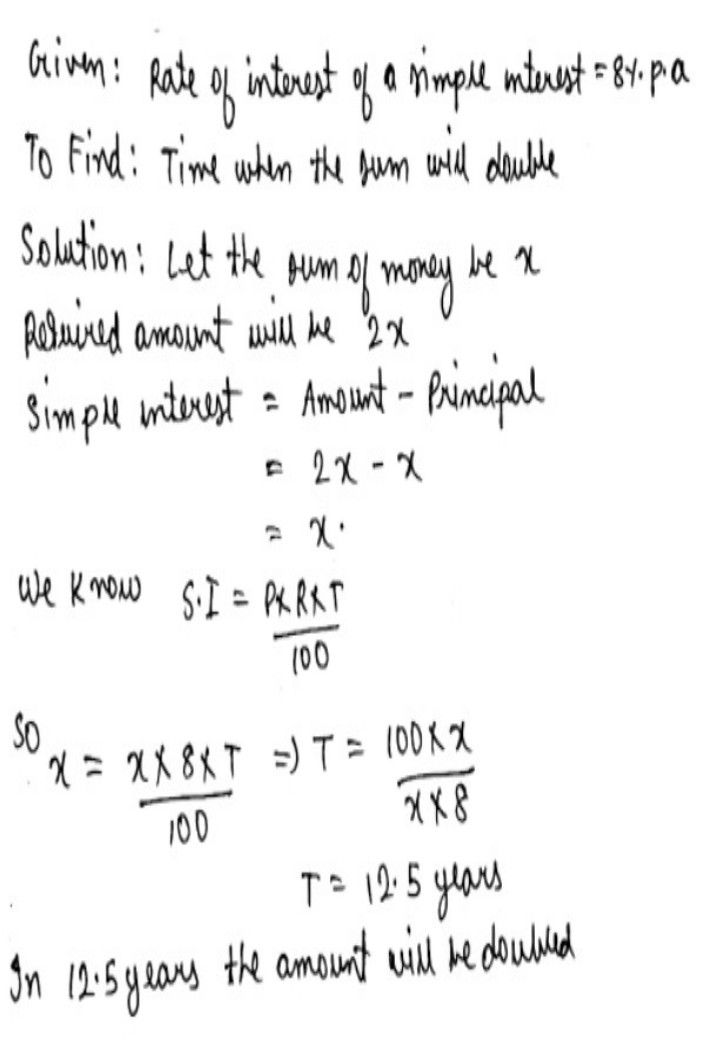Symbol
ProblemIn what time will $a$ sum of money double itself $at$ $8%$ $0$ p.a.
7th-9th grade
Other
SolutionQanda teacher - Bhanusirif you have understand then please evaluate the question gave 5 star⭐ ⭐⭐⭐⭐Student
ok